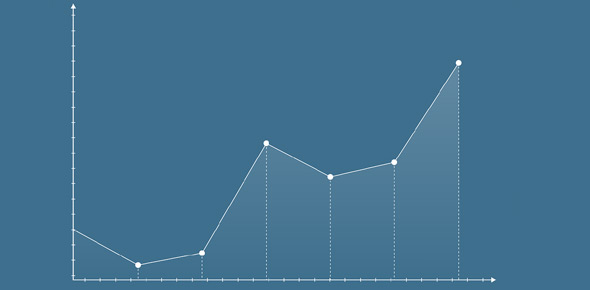2616 Scatter Plot Transformations Reciprocals

16 Questions | Total Attempts: 207Settings1/x (or reciprocal x) and 1/y (or reciprocal y) transformations

• 1.
The 1/y (or reciprocal y) transformation linearises the scatter plot by :
• A.

Turning the y values into 1/y values

• B.

Compressing the y axis values

• C.

Stretching the y axis values

• D.

Leaving the x axis values the same

• 2.
The 1/x transformation linearises the scatter plot by :
• A.

Changing the x values to 1/x values

• B.

Compressing the x axis values

• C.

Stretching the x axis values

• D.

Leaving the y values as they are

• 3.
The scatter plot above will potentially be linearised with :
• A.

1/x transformation

• B.

1/y transformation

• C.

It is is the wrong quadrant for these transformations

• 4.
The scatter plot above will potentially be linearised with :
• A.

1/x transformation

• B.

1/y transformation

• C.

It is is the wrong quadrant for these transformations

• 5.
The scatter plot above will potentially be linearised with :
• A.

1/x transformation

• B.

1/y transformation

• C.

It is is the wrong quadrant for these transformations

• 6.
The scatter plot above will potentially be linearised with :
• A.

1/x transformation

• B.

1/y transformation

• C.

It is is the wrong quadrant for these transformations

• 7.
Which quadrants have potential for a 1/x transformation
• A.

• B.

• C.

• D.

• 8.
Which quadrants have potential for a 1/y transformation
• A.

• B.

• C.

• D.

• 9.
The residual plot above shows the original data is potentially from which quadrants
• A.

• B.

• C.

• D.

• 10.
The residual plot above shows the original data is potentially from which quadrants
• A.

• B.

• C.

• D.

• 11.
The residual plot above shows the original data can potentially be linearised with :
• A.

1/x transformation

• B.

1/y transformation

• C.

It can't be linearised with a reciprocal transformation

• 12.
The residual plot above shows the original data can potentially be linearised with :
• A.

1/x transformation

• B.

1/y transformation

• C.

It can't be linearised with a reciprocal transformation

• 13.
The residual plot above shows the original data can potentially be linearised with :
• A.

1/x transformation

• B.

1/y transformation

• C.

It can't be linearised with a reciprocal transformation

• 14.
The reciprocal transformations are not applicable in which quadrant
• A.

• B.

• C.

• D.

• E.

• 15.
The image above is a transformer from :
• A.

• B.

• C.

• D.Back to top